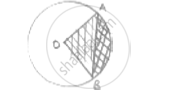Share

# Ab is a Chord of Circle with Centre O and Radius 4cm. Ab is Length of 4cm. Find the Area of Sector of the Circle Formed by Chord Ab - CBSE Class 10 - Mathematics

ConceptAreas of Sector and Segment of a Circle

#### Question

AB is a chord of circle with centre O and radius 4cm. AB is length of 4cm. Find the area of sector of the circle formed by chord AB

#### SolutionAB is chord AB = 4cm

OA = OB = 4cm

OAB is equilateral triangle ∠AOB = 60°

Area of sector (formed by chord [shaded region]) = (area of sector)

=theta/360^@× pir^2 =60/360× pi × 4 × 4 =(8pi)/3 cm^2

Is there an error in this question or solution?

#### APPEARS IN

Solution Ab is a Chord of Circle with Centre O and Radius 4cm. Ab is Length of 4cm. Find the Area of Sector of the Circle Formed by Chord Ab Concept: Areas of Sector and Segment of a Circle.
S